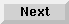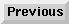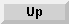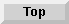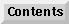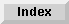5 Operators and Functions

## Binary Operations

Binary operations test and manipulate the individual bits within a single 32-bit word in random access memory. The bit strings referred to in the descriptions are a contiguous series of sixteen bits (1's and 0's) stored in an INTEGER. The rightmost bit (least significant) is designated as bit 0; the leftmost (most significant bit) is bit 31. Operands may be real expressions but must evaluate to a 32-bit signed integer.

BINAND (BINary AND) compares the individual bits in two values which have been rounded to integers. The results are stored in an INTEGER and consist of a 1 in each bit position for which both inputs were 1, and 0's in each bit for which both or either inputs were 0. An error occurs if the values cannot be stored in sixteen bits.

BINAND (numeric expression, numeric expression)

BINIOR (BINary Inclusive OR) compares each bit of two expressions. When a 1 appears in either expression, a 1 is stored in the result. If both bits are 1, a 0 is stored in the result.

BINAND (numeric expression, numeric expression)

BINEOR (BINary Exclusive OR) compares each bit of two expressions. If both bits are 1's or 0's, the result is 0. If only one of the bits is 1, the result is a 1.

BINAND (numeric expression, numeric expression)

BINCMP (BINary CoMPlement) returns a 1 for each bit where the input was a 0, and a 0 in each bit for which the input was a 1 (this gives the complement of the input expression). An error occurs if the rounded numeric expression cannot be stored in an INTEGER.

BINAND (numeric expression, numeric expression)

BIT (Binary Index) contains two expressions. The second expression is evaluated and rounded to an integer which must be in the range 0 to 31. This integer is then used as the index into the binary representation of the integer value of the first expression. Bit 0 is the least significant bit; bit 31 is the most significant bit. The function returns an integer containing the value (0 or 1) found in this position.

An error results if the value of the first expression cannot be rounded to an integer.

BINAND (numeric expression, numeric expression)

ROTATE (binary ROTATE function) evaluates two expressions and rounds them to integers. The first expression is considered a 32-bit word. The function returns the value, as an INTEGER, of the string obtained by rotating the first expression the number of positions specified by the second expression MOD 32. If this second expression is positive, the rotation is toward the least significant bit, and this (least significant) bit is rotated to the most significant bit position. If the second expression is negative, then the rotation is in the opposite direction and bits are transferred from the most to the least significant position. ROTATE does not change the value of the first argument.

An error results if either of the rounded numeric expressions cannot be stored in an integer.

SHIFT (binary SHIFT function) consists of two expressions which are evaluated and rounded to integers. The first expression is considered a 32-bit word which is shifted the number of positions specified by the second expression MOD 32. If the second expression is positive, the shift is toward the least significant bit. If it is negative, the shift is in the opposite direction. Bits which are shifted out are lost and replaced by zeros on the opposite end of the argument. SHIFT does not change the value of its first argument.

An error occurs if either of the rounded numeric expressions cannot be stored in an INTEGER.

SHIFT (numeric expression, numeric expression)

Eloquence Language Manual - 19 DEC 2002